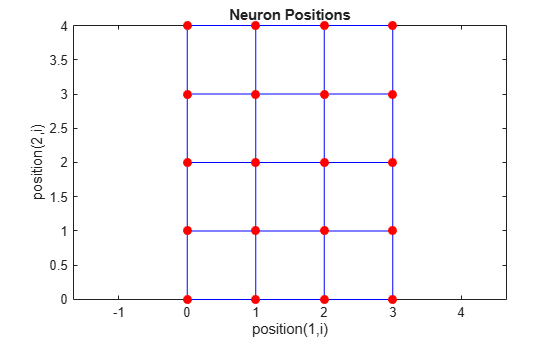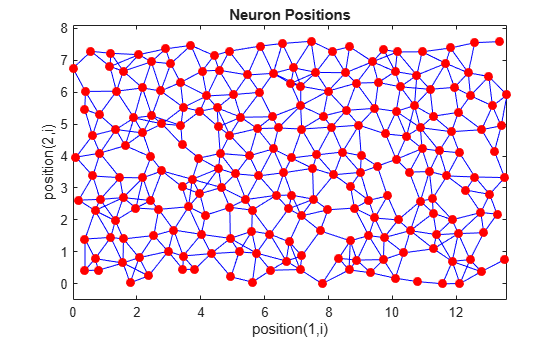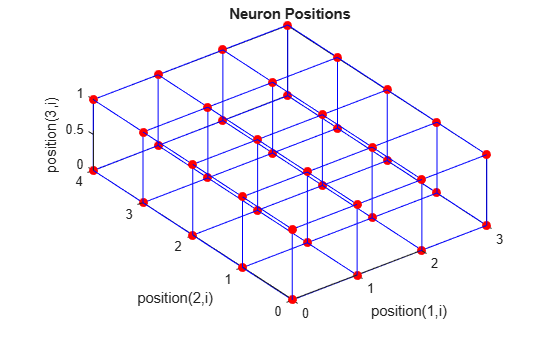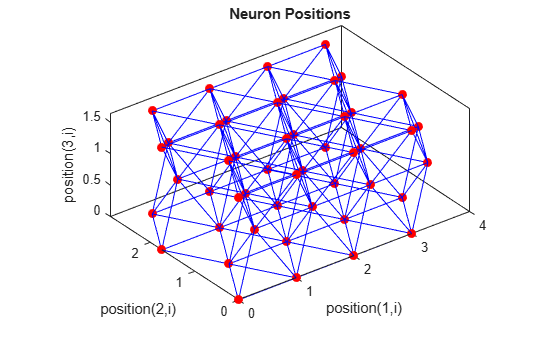# plotsom

Plot self-organizing map

## Syntax

```plotsom(pos) plotsom(W,D,ND) ```

## Description

`plotsom(pos)` takes one argument,

 `POS` `N`-by-`S` matrix of `S` `N`-dimension neural positions

and plots the neuron positions with red dots, linking the neurons within a Euclidean distance of 1.

`plotsom(W,D,ND)` takes three arguments,

 `W` `S`-by-`R` weight matrix `D` `S`-by-`S` distance matrix `ND` Neighborhood distance (default = 1)

and plots the neuron’s weight vectors with connections between weight vectors whose neurons are within a distance of 1.

## Examples

### Plot Self-Organizing Maps

These examples generate plots of various layer topologies.

```pos = hextop([5 6]); plotsom(pos)``````pos = gridtop([4 5]); plotsom(pos)``````pos = randtop([18 12]); plotsom(pos)``````pos = gridtop([4 5 2]); plotsom(pos)``````pos = hextop([4 4 3]); plotsom(pos)```See `plotsompos` for an example of plotting a layer’s weight vectors with the input vectors they map.